13 posts tagged

# Data normalization with SQL

Estimated read time – 5 min

According to GIGO (garbage in, garbage out) principle, errors in input data lead to erroneous analysis results. The results of our work directly depend on the quality of data preparation.

For instance, when we need to prepare data to use in ML algorithms (like k-NN, k-means, logistic regression, etc.), features in the original dataset may vary in scale like the age and height of a person. This may lead to the incorrect performance of the algorithm. Thus, such data needs to be rescaled first.

In this tutorial, we will consider the ways to scale the data using SQL query: min-max normalization, min-max normalization for an arbitrary range, and z-score normalization. For each of these methods we have prepared two SQL query options: one using a SELECT subquery and another using a window function OVER().

We will work with the simple table students that contains the data on the height of the students:

 name height Ivan 174 Peter 181 Dan 199 Kate 158 Mike 179 Silvia 165 Giulia 152 Robert 188 Steven 177 Sophia 165

## Min-max rescaling

Min-max scaling approach scales the data using the fixed range from 0 to 1. In this case, all the data is on the same scale which will exclude the impact of outliers on the conclusions.

The formula for the min-max scaling is given as:

We multiply the numerator by 1.0 in order to get a floating point number at the end.

SQL-query with a subquery:

``````SELECT height,
1.0 * (height-t1.min_height)/(t1.max_height - t1.min_height) AS scaled_minmax
FROM students,
(SELECT min(height) as min_height,
max(height) as max_height
FROM students
) as t1;``````

SQL-query with a window function:

``````SELECT height,
(height - MIN(height) OVER ()) * 1.0 / (MAX(height) OVER () - MIN(height) OVER ()) AS scaled_minmax
FROM students;``````

As a result, we get the values in [0, 1] range where 0 is the height of the shortest student and 1 is the height of the tallest one.

 name height scaled_minmax Ivan 174 0.46809 Peter 181 0.61702 Dan 199 1 Kate 158 0.12766 Mike 179 0.57447 Silvia 165 0.2766 Giulia 152 0 Robert 188 0.76596 Steven 177 0.53191 Sophia 165 0.2766

## Rescaling within a given range

This is an option of min-max normalization between an arbitrary set of values. When it comes to data scaling, the values do not always need to be in the range between 0 and 1. In these cases, the following formula is applied.

This allows us to scale the data to an arbitrary scale. In our example, let’s assume a=10.0 and b=20.0.

SQL-query with subquery:

``````SELECT height,
((height - min_height) * (20.0 - 10.0) / (max_height - min_height)) + 10 AS scaled_ab
FROM students,
(SELECT MAX(height) as max_height,
MIN(height) as min_height
FROM students
) t1;``````

SQL-query with a window function:

``````SELECT height,
((height - MIN(height) OVER() ) * (20.0 - 10.0) / (MAX(height) OVER() - MIN(height) OVER())) + 10.0 AS scaled_ab
FROM students;``````

We receive similar results as before, but with data spread between 10 and 20.

 name height scaled_ab Ivan 174 14.68085 Peter 181 16.17021 Dan 199 20 Kate 158 11.2766 Mike 179 15.74468 Silvia 165 12.76596 Giulia 152 10 Robert 188 17.65957 Steven 177 15.31915 Sophia 165 12.76596

## Z-score normalization

Using Z-score normalization, the data will be scaled so that it has the properties of a standard normal distribution where the mean (μ) is equal to 0 and the standard deviation (σ) to 1.

Z-score is calculated using the formula:

SQL-query with a subquery:

``````SELECT height,
(height - t1.mean) * 1.0 / t1.sigma AS zscore
FROM students,
(SELECT AVG(height) AS mean,
STDDEV(height) AS sigma
FROM students
) t1;``````

SQL-query with a window function:

``````SELECT height,
(height - AVG(height) OVER()) * 1.0 / STDDEV(height) OVER() AS z-score
FROM students;``````

As a result, we can easily notice the outliers that exceed the standard deviation.

 name height zscore Ivan 174 0.01488 Peter 181 0.53582 Dan 199 1.87538 Kate 158 -1.17583 Mike 179 0.38698 Silvia 165 -0.65489 Giulia 152 -1.62235 Robert 188 1.05676 Steven 177 0.23814 Sophia 165 -0.65489
No comments    103   2 mon   Analytics engineering   sql

# How to build Animated Charts like Hans Rosling in Plotly

Estimated read time – 12 min

Hans Rosling’s work on world countries economic growth presented in 2007 at TEDTalks can be attributed to one of the most iconic data visualizations, ever created. Just check out this video, in case you don’t know what we’re talking about:

Sometimes we want to compare standards of living in other countries. One way to do this is to refer to the Big Mac index, which the Economist magazine has kept track of since 1986. The key idea this index represents is to measure purchasing power parity (PPP) in different countries, considering costs of domestic production. To make a standard burger, one would need the following ingredients: cheese, meat, bread and vegetables. Considering that all these ingredients can be produced locally, we can compare the production cost of one Big Mac in different countries, and measure purchasing power. Plus, McDonald’s is the world’s most popular franchise network, its restaurants are almost everywhere around the globe.

In today’s material, we will build a Motion Chart for the Big Mac index using Plotly. Following Hann Rosling’s idea, the chart will display country population along the X-axis and GDP per capita in US dollars along the Y. The size of the dots is going to be proportional to the Big Mac Index for a given country. And the color of the dots will represent the continent where the country is located.

## Preparing Data

Even though The Economist has been updating it for over 30 years and sharing its observations publicly, the dataset contains many missing values. It also lacks continents names, but we can handle it by supplementing the data with some more datasets that can be found in our repo.

Let’s start by importing the libraries:

``````import pandas as pd
from pandas.errors import ParserError
import plotly.graph_objects as go
import numpy as np
import requests
import io``````

We can access the dataset directly from GitHub. Just use the following function to send a GET request to a CSV file and create a Pandas DataFrame. However, in some cases, this may raise a  ParseError because of the caption title, so we will add a try block:

``````def read_raw_file(link):
try:
except ParserError:
return df

From The Economist dataset we will need these columns: country name, local price, dollar exchange rate, country code (iso_a3) and record date. Take the timeline from 2005 to 2020, as the records are most complete for this span. And divide the local price by the exchange rate to calculate the price of one Big Mac in US dollars.

``````bigmac_df = bigmac_df[['name', 'local_price', 'dollar_ex', 'iso_a3', 'date']]
bigmac_df = bigmac_df[bigmac_df['date'] >= '2005-01-01']
bigmac_df = bigmac_df[bigmac_df['date'] < '2020-01-01']
bigmac_df['date'] = pd.DatetimeIndex(bigmac_df['date']).year
bigmac_df = bigmac_df.drop_duplicates(['date', 'name'])
bigmac_df = bigmac_df.reset_index(drop=True)
bigmac_df['dollar_price'] = bigmac_df['local_price'] / bigmac_df['dollar_ex']``````

Take a look at the result:Next, let’s try adding a new column called continents. To ease the task, leave only two columns containing country code and continent name. Then we need to iterate through the bigmac_df[‘iso_a3’] column, adding a continent name for the corresponding values. However some cases may raise an error, because it’s not really clear, whether a country belongs to Europe or Asia, we will consider such cases as Europe by default.

``````continents_df = continents_df[['Continent_Name', 'Three_Letter_Country_Code']]
continents_list = []
for country in bigmac_df['iso_a3']:
try:
continents_list.append(continents_df.loc[continents_df['Three_Letter_Country_Code'] == country]['Continent_Name'].item())
except ValueError:
continents_list.append('Europe')
bigmac_df['continent'] = continents_list``````

Now we can drop unnecessary columns, apply sorting by country names and date, convert values in the date column into integers, and view the current result:

``````bigmac_df = bigmac_df.drop(['local_price', 'iso_a3', 'dollar_ex'], axis=1)
bigmac_df = bigmac_df.sort_values(by=['name', 'date'])
bigmac_df['date'] = bigmac_df['date'].astype(int)``````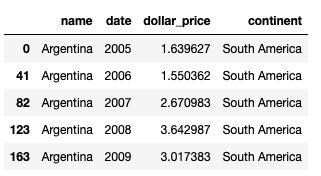Then we need to fill up missing values for The Big Mac index with zeros and remove the Republic of China, since this partially recognized state is not included in the World Bank datasets. The UAE occurs several times, this can lead to issues.

``````countries_list = list(bigmac_df['name'].unique())
years_set = {i for i in range(2005, 2020)}
for country in countries_list:
if len(bigmac_df[bigmac_df['name'] == country]) < 15:
this_continent = bigmac_df[bigmac_df['name'] == country].continent.iloc
years_of_country = set(bigmac_df[bigmac_df['name'] == country]['date'])
diff = years_set - years_of_country
dict_to_df = pd.DataFrame({
'name':[country] * len(diff),
'date':list(diff),
'dollar_price': * len(diff),
'continent': [this_continent] * len(diff)
})
bigmac_df = bigmac_df.append(dict_to_df)
bigmac_df = bigmac_df[bigmac_df['name'] != 'Taiwan']
bigmac_df = bigmac_df[bigmac_df['name'] != 'United Arab Emirates']``````

Next, let’s augment the data with GDP per capita and population from other datasets. Both datasets have differences in country names, so we need to specify such cases explicitly and replace them.

``````years = [str(i) for i in range(2005, 2020)]

countries_replace_dict = {
'Russian Federation': 'Russia',
'Egypt, Arab Rep.': 'Egypt',
'Hong Kong SAR, China': 'Hong Kong',
'United Kingdom': 'Britain',
'Korea, Rep.': 'South Korea',
'United Arab Emirates': 'UAE',
'Venezuela, RB': 'Venezuela'
}
for key, value in countries_replace_dict.items():
population_df['Country Name'] = population_df['Country Name'].replace(key, value)
gdp_df['Country Name'] = gdp_df['Country Name'].replace(key, value)``````

Finally, extract population data and GDP for the given years, adding the data to the bigmac_df DataFrame:

``````countries_list = list(bigmac_df['name'].unique())

population_list = []
gdp_list = []
for country in countries_list:
population_for_country_df = population_df[population_df['Country Name'] == country][years]
population_list.extend(list(population_for_country_df.values))
gdp_for_country_df = gdp_df[gdp_df['Country Name'] == country][years]
gdp_list.extend(list(gdp_for_country_df.values))

bigmac_df['population'] = population_list
bigmac_df['gdp'] = gdp_list
bigmac_df['gdp_per_capita'] = bigmac_df['gdp'] / bigmac_df['population']``````

And here is our final dataset: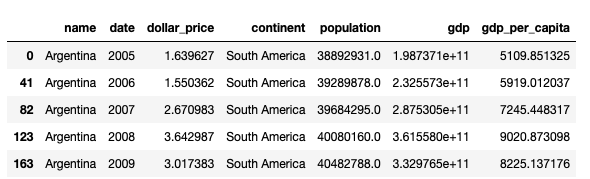## Creating a chart in Plotly

The population in China or India, on average, is 10 times more than in other countries. That’s why we need to transform X-axis to Log Scale, to make the chart easier for interpreting. The log-transformation is a common way to address skewness in data.

``````fig_dict = {
"data": [],
"layout": {},
"frames": []
}

fig_dict["layout"]["xaxis"] = {"title": "Population", "type": "log"}
fig_dict["layout"]["yaxis"] = {"title": "GDP per capita (in \$)", "range":[-10000, 120000]}
fig_dict["layout"]["hovermode"] = "closest"
{
"buttons": [
{
"args": [None, {"frame": {"duration": 500, "redraw": False},
"fromcurrent": True, "transition": {"duration": 300,
"label": "Play",
"method": "animate"
},
{
"args": [[None], {"frame": {"duration": 0, "redraw": False},
"mode": "immediate",
"transition": {"duration": 0}}],
"label": "Pause",
"method": "animate"
}
],
"direction": "left",
"showactive": False,
"type": "buttons",
"x": 0.1,
"xanchor": "right",
"y": 0,
"yanchor": "top"
}
]``````

We will also add a slider to filter data within a certain range:

``````sliders_dict = {
"active": 0,
"yanchor": "top",
"xanchor": "left",
"currentvalue": {
"font": {"size": 20},
"prefix": "Year: ",
"visible": True,
"xanchor": "right"
},
"transition": {"duration": 300, "easing": "cubic-in-out"},
"len": 0.9,
"x": 0.1,
"y": 0,
"steps": []
}``````

By default, the chart will display data for 2005 before we click on the “Play” button.

``````continents_list_from_df = list(bigmac_df['continent'].unique())
year = 2005
for continent in continents_list_from_df:
dataset_by_year = bigmac_df[bigmac_df["date"] == year]
dataset_by_year_and_cont = dataset_by_year[dataset_by_year["continent"] == continent]

data_dict = {
"x": dataset_by_year_and_cont["population"],
"y": dataset_by_year_and_cont["gdp_per_capita"],
"mode": "markers",
"text": dataset_by_year_and_cont["name"],
"marker": {
"sizemode": "area",
"sizeref": 200000,
"size":  np.array(dataset_by_year_and_cont["dollar_price"]) * 20000000
},
"name": continent,
"customdata": np.array(dataset_by_year_and_cont["dollar_price"]).round(1),
"hovertemplate": '<b>%{text}</b>' + '<br>' +
'GDP per capita: %{y}' + '<br>' +
'Population: %{x}' + '<br>' +
'Big Mac price: %{customdata}\$' +
'<extra></extra>'
}
fig_dict["data"].append(data_dict)``````

Next, we need to fill up the frames field, which will be used for animating the data. Each frame represents a certain data point from 2005 to 2019.

``````for year in years:
frame = {"data": [], "name": str(year)}
for continent in continents_list_from_df:
dataset_by_year = bigmac_df[bigmac_df["date"] == int(year)]
dataset_by_year_and_cont = dataset_by_year[dataset_by_year["continent"] == continent]

data_dict = {
"x": list(dataset_by_year_and_cont["population"]),
"y": list(dataset_by_year_and_cont["gdp_per_capita"]),
"mode": "markers",
"text": list(dataset_by_year_and_cont["name"]),
"marker": {
"sizemode": "area",
"sizeref": 200000,
"size": np.array(dataset_by_year_and_cont["dollar_price"]) * 20000000
},
"name": continent,
"customdata": np.array(dataset_by_year_and_cont["dollar_price"]).round(1),
"hovertemplate": '<b>%{text}</b>' + '<br>' +
'GDP per capita: %{y}' + '<br>' +
'Population: %{x}' + '<br>' +
'Big Mac price: %{customdata}\$' +
'<extra></extra>'
}
frame["data"].append(data_dict)

fig_dict["frames"].append(frame)
slider_step = {"args": [
[year],
{"frame": {"duration": 300, "redraw": False},
"mode": "immediate",
"transition": {"duration": 300}}
],
"label": year,
"method": "animate"}
sliders_dict["steps"].append(slider_step)``````

Just a few finishing touches left, instantiate the chart, set colors, fonts and title.

``````fig_dict["layout"]["sliders"] = [sliders_dict]

fig = go.Figure(fig_dict)

fig.update_layout(
title =
{'text':'<b>Motion chart</b><br><span style="color:#666666">The Big Mac index from 2005 to 2019</span>'},
font={
'family':'Open Sans, light',
'color':'black',
'size':14
},
plot_bgcolor='rgba(0,0,0,0)'
)
fig.update_yaxes(nticks=4)
fig.update_xaxes(tickfont=dict(family='Open Sans, light', color='black', size=12), nticks=4, gridcolor='lightgray', gridwidth=0.5)
fig.update_yaxes(tickfont=dict(family='Open Sans, light', color='black', size=12), nticks=4, gridcolor='lightgray', gridwidth=0.5)

fig.show()``````

Bingo! The Motion Chart is done:

View the code on GitHub

No comments    266   9 mon   Analytics engineering   data analytics   plotly

# Collecting Social Media Data for Top ML, AI & Data Science related accounts on Instagram

Estimated read time – 9 min

Instagram is in the top 5 most visited websites, perhaps not for our industry. Nevertheless, we are going to test this hypothesis using Python and our data analytics skills. In this post, we will share how to collect social media data using the Instagram API.

Data collection method
The Instagram API won’t let us collect data about other platform users for no reason, but there is always a way. Try sending the following request:

``https://instagram.com/leftjoin/?__a=1``

The request returns a JSON object with detailed user information, for instance, we can easily get an account name, number of posts, followers, subscriptions, as well as the first ten user posts with likes count, comments and etc. The pyInstagram library allows sending such requests.

SQL schema
Data will be collected into thee Clickhouse tables: users, posts, comments. The users table will contain user data, such as user id, username, user’s first and last name, account description, number of followers, subscriptions, posts, comments, and likes, whether an account is verified or not, and so on.

``````CREATE TABLE instagram.users
(
`user_id` UInt64,
`user_name` String,
`full_name` String,
`base_url` String,
`biography` String,
`followers_count` UInt64,
`follows_count` UInt64,
`media_count` UInt64,
`total_likes` UInt64,
`is_verified` UInt8,
`country_block` UInt8,
`profile_pic_url` Nullable(String),
`profile_pic_url_hd` Nullable(String),
`fb_page` Nullable(String)
)
ENGINE = ReplacingMergeTree

The posts table will be populated with the post owner name, post id, caption, comments coun, and so on. To check whether a post is an advertisement, Instagram carousel, or a video we can use these fields: is_ad, is_album and is_video.

``````CREATE TABLE instagram.posts
(
`owner` String,
`post_id` UInt64,
`caption` Nullable(String),
`code` String,
`created_at` DateTime,
`display_url` String,
`is_album` UInt8,
`is_video` UInt8,
`likes_count` UInt64,
`location` Nullable(String),
`recources` Array(String),
`video_url` Nullable(String)
)
ENGINE = ReplacingMergeTree

In the comments table, we store each comment separately with the comment owner and text.

``````CREATE TABLE instagram.comments
(
`comment_id` UInt64,
`post_id` UInt64,
`comment_owner` String,
`comment_text` String
)
ENGINE = ReplacingMergeTree

Writing the script
Import the following classes from the library: Account, Media, WebAgent and Comment.

``````from instagram import Account, Media, WebAgent, Comment
from datetime import datetime
from clickhouse_driver import Client
import requests
import pandas as pd``````

Next, create an instance of the WebAgent class required for some library methods and data updating. To collect any meaningful information we need to have at least account names. Since we don’t have them yet, send the following request to search for porifles by the  keywords specified in queries_list. The search results will be composed of Instagram pages that match any keyword in the list.

``````agent = WebAgent()
queries_list = ['machine learning', 'data science', 'data analytics', 'analytics', 'business intelligence',
'data engineering', 'computer science', 'big data', 'artificial intelligence',
'deep learning', 'data scientist','machine learning engineer', 'data engineer']
client = Client(host='12.34.56.789', user='default', password='', port='9000', database='instagram')
url = 'https://www.instagram.com/web/search/topsearch/?context=user&count=0'``````

Let’s iterate the keywords collecting all matching accounts. Then remove duplicates from the obtained list by converting it to set and back.

``````response_list = []
for query in queries_list:
response = requests.get(url, params={
'query': query
}).json()
response_list.extend(response['users'])
instagram_pages_list = []
for item in response_list:
instagram_pages_list = list(set(instagram_pages_list))``````

Now we need to loop through the list of pages and request detailed information about an account if it’s not in the table yet. Create an instance of the Account class and pass username as a parameter.
Then update the account information using the agent.update()
method. We will collect only the first 100 posts to keep it moving. Next, create a list named media_list to store received post ids after calling the agent.get_media() method.

Collecting user media data

``````all_posts_list = []
if client.execute(f"SELECT count(1) FROM users WHERE user_name='{username}'") == 0:
account_total_likes = 0
try:
except Exception as E:
print(E)
continue
try:
agent.update(account)
except Exception as E:
print(E)
continue
if account.media_count < 100:
post_count = account.media_count
else:
post_count = 100
print(account, post_count)
media_list, _ = agent.get_media(account, count=post_count, delay=1)
count = 0``````

Because we need to count the total number of likes and comments before adding a new user to our database, we’ll start with them first. Almost all required fields belong to the Media class:

Collecting user posts

``````for media_code in media_list:
if client.execute(f"SELECT count(1) FROM posts WHERE code='{media_code}'") == 0:
print('posts:', count, '/', len(media_list))
count += 1

post_insert_list = []
post = Media(media_code)
agent.update(post)
post_insert_list.append(datetime.now().strftime('%Y-%m-%d %H:%M:%S'))
post_insert_list.append(str(post.owner))
post_insert_list.append(post.id)
if post.caption is not None:
post_insert_list.append(post.caption.replace("'","").replace('"', ''))
else:
post_insert_list.append("")
post_insert_list.append(post.code)
post_insert_list.append(datetime.fromtimestamp(post.date).strftime('%Y-%m-%d %H:%M:%S'))
post_insert_list.append(post.display_url)
try:
except TypeError:
post_insert_list.append('cast(Null as Nullable(UInt8))')
post_insert_list.append(int(post.is_album))
post_insert_list.append(int(post.is_video))
post_insert_list.append(post.likes_count)
if post.location is not None:
post_insert_list.append(post.location)
else:
post_insert_list.append('')
post_insert_list.append(post.resources)
if post.video_url is not None:
post_insert_list.append(post.video_url)
else:
post_insert_list.append('')
account_total_likes += post.likes_count
try:
client.execute(f'''
INSERT INTO posts VALUES {tuple(post_insert_list)}
''')
except Exception as E:
print('posts:')
print(E)
print(post_insert_list)``````

Store comments in the variable with the same name after calling the get_comments() method:

``````comments = agent.get_comments(media=post)
comment_insert_list = []
comment = Comment(comment_id)
comment_insert_list.append(datetime.now().strftime('%Y-%m-%d %H:%M:%S'))
comment_insert_list.append(comment.id)
comment_insert_list.append(post.id)
comment_insert_list.append(str(comment.owner))
comment_insert_list.append(comment.text.replace("'","").replace('"', ''))
try:
client.execute(f'''
''')
except Exception as E:
print(E)
print(comment_insert_list)``````

And now, when we have obtained user posts and comments new information can be added to the table.

Collecting user data

``````user_insert_list = []
user_insert_list.append(datetime.now().strftime('%Y-%m-%d %H:%M:%S'))
user_insert_list.append(account.id)
user_insert_list.append(account.full_name)
user_insert_list.append(account.base_url)
user_insert_list.append(account.biography)
user_insert_list.append(account.followers_count)
user_insert_list.append(account.follows_count)
user_insert_list.append(account.media_count)
user_insert_list.append(account_total_likes)
user_insert_list.append(int(account.is_verified))
user_insert_list.append(int(account.country_block))
user_insert_list.append(account.profile_pic_url)
user_insert_list.append(account.profile_pic_url_hd)
if account.fb_page is not None:
user_insert_list.append(account.fb_page)
else:
user_insert_list.append('')
try:
client.execute(f'''
INSERT INTO users VALUES {tuple(user_insert_list)}
''')
except Exception as E:
print('users:')
print(E)
print(user_insert_list)``````

Conclusion
To sum up, we have collected data of 500 users, with nearly 20K posts and 40K comments. As the database will be updated, we can write a simple query to get the top 10 ML, AI & Data Science related most followed accounts for today.

``````SELECT *
FROM users
ORDER BY followers_count DESC
LIMIT 10``````

And as a bonus, here is a list of the most interesting Instagram accounts on this topic:

View the code on GitHub

No comments    235   10 mon   Analytics engineering   clickhouse   data analytics   instagram   python

# Analyzing Business Intelligence (BI) and Analytics job market in Tableau

Estimated read time – 13 min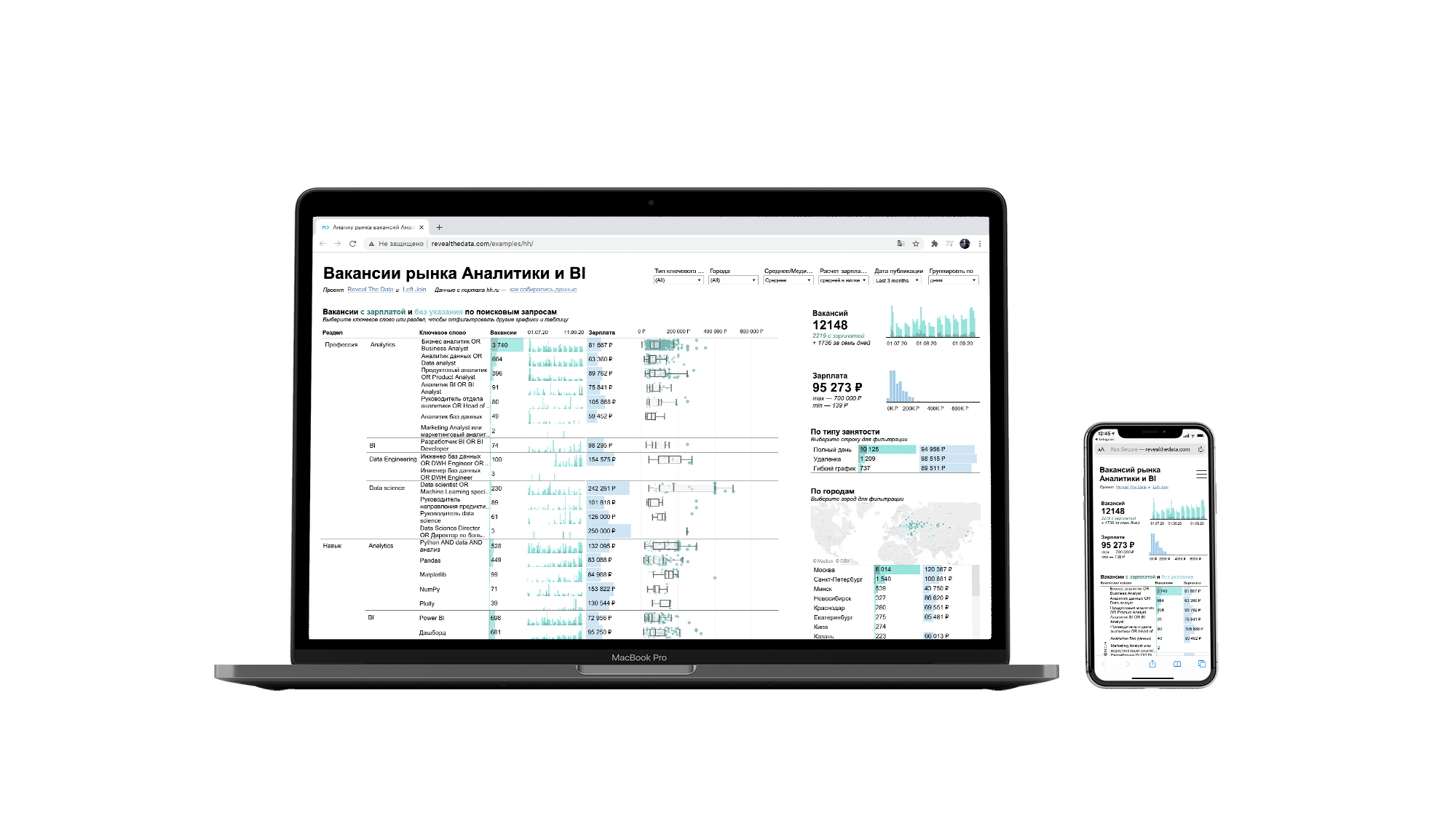According to the SimilarWeb rating, hh.ru is the third among the most popular job search websites in the world. In one of the conversations with Roman Bunin, we came up with the idea of making a common project and collect data using the HeadHunter API for later analysis and visualization in Tableau Public. Our goal was to understand the dependency between salary and skills specified in a job posting and compare how things are in Moscow, Saint Petersburg, and other regions.

## Data Collection Process

Our scheme is based on fetching a  brief job description, returned by the GET /vacancies method. According to the structure we need to create the following columns: vacancy type, id, vacancy rate (‘premium’), pre-employment testing (‘has_test’), company address, salary, work schedule, and so forth. We created a table using the following CREATE query down below:

Query for creating the vacancies_short table in ClickHouse

``````CREATE TABLE headhunter.vacancies_short
(
`query_string` String,
`type` String,
`level` String,
`direction` String,
`vacancy_id` UInt64,
`has_test` UInt8,
`response_url` String,
`alternate_url` String,
`apply_alternate_url` String,
`department_id` String,
`department_name` String,
`salary_from` Nullable(Float64),
`salary_to` Nullable(Float64),
`salary_currency` String,
`salary_gross` Nullable(UInt8),
`name` String,
`insider_interview_id` Nullable(UInt64),
`insider_interview_url` String,
`area_url` String,
`area_id` UInt64,
`area_name` String,
`url` String,
`published_at` DateTime,
`employer_url` String,
`employer_alternate_url` String,
`employer_logo_urls_90` String,
`employer_logo_urls_240` String,
`employer_logo_urls_original` String,
`employer_name` String,
`employer_id` UInt64,
`response_letter_required` UInt8,
`type_id` String,
`type_name` String,
`archived` UInt8,
`schedule_id` Nullable(String)
)
ENGINE = ReplacingMergeTree
ORDER BY vacancy_id``````

The first script collects data from the HeadHunter website through API and inserts to our Database using the following libraries:

``````import requests
from clickhouse_driver import Client
from datetime import datetime
import pandas as pd
import re``````

Next, we create a DataFrame and connect to the Database in ClickHouse:

``````queries = pd.read_csv('hh_data.csv')

The queries table stores a list of our search queries, having the following columns: query type, level, career field, and search phrase. The last column contains logical operators, for instance, we can get more results by putting logical ANDs between “Python”, “data” and “analysis”.The search results may not always match the expectations, chiefs, marketers, and administrators can accidentally get into our database. To prevent this, we will write a function named check_name(name), it will accept a vacancy name and return a boolean value, depending on the match.

``````def check_name(name):
bad_names = [r'курьер', r'грузчик', r'врач', r'менеджер по закупу',
r'менеджер по продажам', r'оператор', r'повар', r'продавец',
r'директор магазина', r'директор по продажам', r'директор по маркетингу',
r'кабельщик', r'начальник отдела продаж', r'заместитель', r'администратор магазина',
r'категорийный', r'аудитор', r'юрист', r'контент', r'супервайзер', r'стажер-ученик',
r'су-шеф', r'маркетолог\$', r'региональный', r'ревизор', r'экономист', r'ветеринар',
r'торговый', r'клиентский', r'начальник цеха', r'территориальный', r'переводчик',
r'маркетолог /', r'маркетолог по']
if re.match(item, name):
return True``````

Moving further, we need to create a while loop to collect data non-stop. Iterate over the Dataframe queries selecting the type, level, field, and search phrase columns. Send a GET request using a keyword to get the number of pages. Then we loop through the number of pages sending the same requests and populating vacancies_from_response with job descriptions. In the per_page parameter we specified 10, this is the max limit for the HH API. Since we didn’t pass any value to the area field, the results are collected worldwide.

``````while True:
for query_type, level, direction, query_string in zip(queries['Query Type'], queries['Level'], queries['Career Field'], queries['Seach Phrase']):
print(f'seach phrase: {query_string}')
url = 'https://api.hh.ru/vacancies'
par = {'text': query_string, 'per_page':'10', 'page':0}
r = requests.get(url, params=par).json()
pages = r['pages']
found = r['found']
vacancies_from_response = []

for i in range(0, pages + 1):
par = {'text': query_string, 'per_page':'10', 'page':i}
r = requests.get(url, params=par).json()
try:
vacancies_from_response.append(r['items'])
except Exception as E:
continue``````

Create a for loop to escape duplicate rows in our table. First, send a query to the database, verifying whether there is a vacancy with the same id and search phrase. If the verification was successful we then
pass the job title to check_name() and move on to the next one.

``````for item in vacancies_from_response:
for vacancy in item:
if client.execute(f"SELECT count(1) FROM vacancies_short WHERE vacancy_id={vacancy['id']} AND query_string='{query_string}'") == 0:
name = vacancy['name'].replace("'","").replace('"','')
if check_name(name):
continue``````

Now we need to extract all the necessary data from a job description. The table will contain empty cells, since some data may be missing.

View the code for extracting job description data

``````vacancy_id = vacancy['id']
has_test = int(vacancy['has_test'])
response_url = vacancy['response_url']
try:
except TypeError:
alternate_url = vacancy['alternate_url']
apply_alternate_url = vacancy['apply_alternate_url']
try:
department_id = vacancy['department']['id']
except TypeError as E:
department_id = ""
try:
department_name = vacancy['department']['name']
except TypeError as E:
department_name = ""
try:
salary_from = vacancy['salary']['from']
except TypeError as E:
salary_from = "cast(Null as Nullable(UInt64))"
try:
salary_to = vacancy['salary']['to']
except TypeError as E:
salary_to = "cast(Null as Nullable(UInt64))"
try:
salary_currency = vacancy['salary']['currency']
except TypeError as E:
salary_currency = ""
try:
salary_gross = int(vacancy['salary']['gross'])
except TypeError as E:
salary_gross = "cast(Null as Nullable(UInt8))"
try:
insider_interview_id = vacancy['insider_interview']['id']
except TypeError:
insider_interview_id = "cast(Null as Nullable(UInt64))"
try:
insider_interview_url = vacancy['insider_interview']['url']
except TypeError:
insider_interview_url = ""
area_url = vacancy['area']['url']
area_id = vacancy['area']['id']
area_name = vacancy['area']['name']
url = vacancy['url']
published_at = vacancy['published_at']
published_at = datetime.strptime(published_at,'%Y-%m-%dT%H:%M:%S%z').strftime('%Y-%m-%d %H:%M:%S')
try:
employer_url = vacancy['employer']['url']
except Exception as E:
print(E)
employer_url = ""
try:
employer_alternate_url = vacancy['employer']['alternate_url']
except Exception as E:
print(E)
employer_alternate_url = ""
try:
employer_logo_urls_90 = vacancy['employer']['logo_urls']['90']
employer_logo_urls_240 = vacancy['employer']['logo_urls']['240']
employer_logo_urls_original = vacancy['employer']['logo_urls']['original']
except Exception as E:
print(E)
employer_logo_urls_90 = ""
employer_logo_urls_240 = ""
employer_logo_urls_original = ""
employer_name = vacancy['employer']['name'].replace("'","").replace('"','')
try:
employer_id = vacancy['employer']['id']
except Exception as E:
print(E)
response_letter_required = int(vacancy['response_letter_required'])
type_id = vacancy['type']['id']
type_name = vacancy['type']['name']
is_archived = int(vacancy['archived'])``````

The last field is the work schedule. If there is mentioned a fly-in-fly-out method, these kinds of job postings will be skipped.

``````try:
schedule = vacancy['schedule']['id']
except Exception as E:
print(E)
schedule = ''"
if schedule == 'flyInFlyOut':
continue``````

Next, we create a list of obtained variables, replacing None values with empty strings to escape errors with Clickhouse and insert them into the table.

``````vacancies_short_list = [added_at, query_string, query_type, level, direction, vacancy_id, is_premium, has_test, response_url, address_city, address_street, address_building, address_description, address_lat, address_lng, address_raw, address_metro_stations, alternate_url, apply_alternate_url, department_id, department_name,
salary_from, salary_to, salary_currency, salary_gross, insider_interview_id, insider_interview_url, area_url, area_name, url, published_at, employer_url, employer_logo_urls_90, employer_logo_urls_240,  employer_name, employer_id, response_letter_required, type_id, type_name, is_archived, schedule]
for index, item in enumerate(vacancies_short_list):
if item is None:
vacancies_short_list[index] = ""
tuple_to_insert = tuple(vacancies_short_list)
print(tuple_to_insert)
client.execute(f'INSERT INTO vacancies_short VALUES {tuple_to_insert}')``````

## Connecting Tableau to the data source

Unfortunately, we can’t work with databases in  Tableau Public, that’s why we decided to connect our Clickhouse Database to Google Sheets. With this in mind, we picked the following libraries: gspread and oauth2client for accessing Google Spreadsheets API, and schedule for task scheduling.

Refer to our previous article where we used  Google Spreadseets API for  Collecting Data on Ad Campaigns from VK.com

``````import schedule
from clickhouse_driver import Client
import pandas as pd
from oauth2client.service_account import ServiceAccountCredentials
from datetime import datetime

creds = ServiceAccountCredentials.from_json_keyfile_name('credentials.json', scope)

The update_sheet() function will transfer all data from Clickhouse to a Google Sheets table:

``````def update_sheet():
print('Updating cell at', datetime.now())
columns = []
for item in client.execute('describe table headhunter.vacancies_short'):
columns.append(item)
vacancies = client.execute('SELECT * FROM headhunter.vacancies_short')
df_vacancies = pd.DataFrame(vacancies, columns=columns)
df_vacancies.to_csv('vacancies_short.csv', index=False)
gc.import_csv('1ZWS2kqraPa4i72hzp0noU02SrYVo0teD7KZ0c3hl-UI', content.encode('utf-8'))``````

Using schedule to run our function every day at 1:00 PM (UTC):

``````schedule.every().day.at("13:00").do(update_sheet)
while True:
schedule.run_pending()``````

## What’s the final point?

Roman created an informative dashboard based on this data.

And made a youtube video with a detailed explanation of the dashboard features.

## Key Insights

1. Data Analysts specializing in BI are most in-demand in the job market since the highest number of search results were returned with this query. However, the average salary is higher in Product Analyst and BI-analyst openings.
2. Most of the postings were found In Moscow, where the average salary is 10-30K RUB higher than in Saint Petersburg and 30-40K higher than in other regions.
3. Top highly paid positions: Head of Analytics (110K RUB per month on avg.), Database Engineer (138K RUB per month), and Head of Machine Learning (250K RUB per month).
4. The most useful skills to have are a solid knowledge of Python with Pandas and Numpy, Tableau, Power BI, ETL, and Spark. Most of the posings found contained these requirements and were highly paid than any others. For Python programmers, it’s more valuable to have expertise with Matplotlib than Plotly.

View the code on  GitHub

# Sentiment analysis of Russians on Constitutional Amendments

Estimated read time – 11 min

In today’s article, we are going to use public data from vk.com to interpret and classify users’ attitudes about the 2020 amendments to the Constitution of Russia.

## API Overview

First off, we need to receive data using the newsfeed.search method, this method allows us to get up to one thousand of the latest posts from the news feed by keyword.
The response data contains different fields, like post ids, user or community ids, text data, likes count, comments, apps, geolocation, and many more. We are only needed ids and text data.
Some expanded information about the author will also be useful for our analysis, this includes city, gender, age, and can be received with the users.get method.

## Create Clickhouse Tables

The received data should be stored somewhere, we chose to use ClickHouse, an open-source column-oriented DBMS. Let’s create two tables to store users and their posts. The first table will be populated with ids and text data, the second one will hold user data, such as their ids, age, and city. The ReplacingMergeTree () engine will remove duplicates in our tables.

The article assumes that you’re familiar with how to install ClickHouse on AWS, create external dictionaries and  materialized views

``````CREATE TABLE vk_posts(
post_id UInt64,
post_date DateTime,
owner_id UInt64,
from_id UInt64,
text String
) ENGINE ReplacingMergeTree()
ORDER BY post_date

CREATE TABLE vk_users(
user_id UInt64,
user_sex Nullable(UInt8),
user_city String,
user_age Nullable(UInt16)
) ENGINE ReplacingMergeTree()
ORDER BY user_id``````

## Collecting user posts with the VK API

Let’s get to writing our script, import the libraries, and create several variables with constant values:

If you don’t have an access token yet and want to create one, refer to this step by step guide: “Collecting Data on Ad Campaigns from VK.com”

``````from clickhouse_driver import Client
from datetime import datetime
import requests
import pandas as pd
import time

token = 'your_token'
version = 5.103
client = Client(host='ec1-23-456-789-1011.us-east-2.compute.amazonaws.com', user='default', password='', port='9000', database='default')
data_list = []
start_from = 0
query_string = 'конституция' #constitution``````

Define the get_and_insert_info_by_user function that will receive a list of user ids and expanded information about them, and send it to the vk_users table. Since the user_ids parameter takes a list as a string object, we need to change the structure and omit the square brackets.
Most users prefer to conceal their gender, age, and city. In such cases, we need to use Nullable values. To obtain user age we need to subtract the birth year from the current year, if the birth year is missing we can check it using the regular expression.

get_and_insert_info_by_user() function

``````def get_and_insert_info_by_user(users):
try:
r = requests.get('https://api.vk.com/method/users.get', params={
'access_token':token,
'v':version,
'user_ids':str(users)[1:-2],
'fields':'sex, city, bdate'
}).json()['response']
for user in r:
user_list = []
user_list.append(user['id'])
if client.execute(f"SELECT count(1) FROM vk_users where user_id={user['id']}") == 0:
print(user['id'])
try:
user_list.append(user['sex'])
except Exception:
user_list.append('cast(Null as Nullable(UInt8))')
try:
user_list.append(user['city']['title'])
except Exception:
user_list.append('')
try:
now = datetime.now()
year = item.split('.')[-1]
if re.match(r'\d\d\d\d', year):
age = now.year - int(year)
user_list.append(age)
except Exception:
user_list.append('cast(Null as Nullable(UInt16))')
user_insert_tuple = tuple(user_list)
client.execute(f'INSERT INTO vk_users VALUES {user_insert_tuple}')
except KeyError:
pass``````

Our script will work in a while loop to constantly update data, as we can only receive a thousand of the latest data points.The newsfeed.search method returns 200 posts per call, so we need to invoke it five times to collect all the posts.

While loop to collect new posts

``````while True:
for i in range(5):
r = requests.get('https://api.vk.com/method/newsfeed.search', params={
'access_token':token,
'v':version,
'q':query_string,
'count':200,
'start_from': start_from
})
data_list.append(r.json()['response'])
try:
start_from = r.json()['response']['next_from']
except KeyError:
pass``````

The data we received can be parsed, VK users always have a positive id, while for communities it’s negative. We need only users data for our analysis, where from_id > 0. The next step is to check whether a post contains any text data or not. Finally, we will collect and store unique entries by user id. Pause the script after each iteration for 180 seconds to wait for new user posts and not violate the VK API rules.

``````user_ids = []
for data in data_list:
for data_item in data['items']:
if data_item['from_id'] > 0:
post_list = []
if not data_item['text']:
continue
if client.execute(f"SELECT count(1) FROM vk_posts WHERE post_id={data_item['id']} AND from_id={data_item['from_id']}") == 0:
user_ids.append(data_item['from_id'])
date = datetime.fromtimestamp(data_item['date'])
date = datetime.strftime(date, '%Y-%m-%d %H:%M:%S')
post_list.append(date)
post_list.append(data_item['id'])
post_list.append(data_item['owner_id'])
post_list.append(data_item['from_id'])
post_list.append(data_item['text'].replace("'","").replace('"','').replace("\n",""))
post_list.append(query_string)
post_tuple = tuple(post_list)
print(post_list)
try:
client.execute(f'INSERT INTO vk_posts VALUES {post_tuple}')
except Exception as E:
print('!!!!! try to insert into vk_post but got', E)
try:
get_and_insert_info_by_user(user_ids)
except Exception as E:
print("Try to insert user list:", user_ids, "but got:", E)
time.sleep(180)``````

## Dostoevsky for sentiment analysis

For one week our script collected almost 20000 posts from VK users that mention the keyword “constitution” (or “конституция” in Russian). It’s time to write our second script for data analysis and visualization. First, create a DataFrame with the data received, and evaluate the sentiment of each post, identifying whether it’s positive, negative, or neutral. We are going to use the Dostoevsky library to analyze the emotion behind a text.

``````from dostoevsky.tokenization import RegexTokenizer
from dostoevsky.models import FastTextSocialNetworkModel
from clickhouse_driver import Client
import pandas as pd
client = Client(host='ec1-23-456-789-1011.us-east-2.compute.amazonaws.com', user='default', password='', port='9000', database='default')``````

Assign all the contents of our table to the vk_posts variable with a simple query. Iterate through all the posts, select those with text data and populate our DataFrame.

``````vk_posts = client.execute('SELECT * FROM vk_posts')
list_of_posts = []
list_of_ids = []
for post in vk_posts:
if str(post[-2]).replace(" ", ""):
list_of_posts.append(str(post[-2]).replace("\n",""))
list_of_ids.append(int(post))
df_posts = pd.DataFrame()
df_posts['post'] = list_of_posts
df_posts['id'] = list_of_ids``````

Instantiate our model and iterate through the posts to evaluate the sentiment of each entry.

``````tokenizer = RegexTokenizer()
model = FastTextSocialNetworkModel(tokenizer=tokenizer)
sentiment_list = []
results = model.predict(list_of_posts, k=2)
for sentiment in results:
sentiment_list.append(sentiment)``````

Add several boolean columns to our DataFrame that will reflect whether it’s a  positive, negative, or neutral post.

``````neutral_list = []
negative_list = []
positive_list = []
speech_list = []
skip_list = []
for sentiment in sentiment_list:
neutral = sentiment.get('neutral')
negative = sentiment.get('negative')
positive = sentiment.get('positive')
if neutral is None:
neutral_list.append(0)
else:
neutral_list.append(sentiment.get('neutral'))
if negative is None:
negative_list.append(0)
else:
negative_list.append(sentiment.get('negative'))
if positive is None:
positive_list.append(0)
else:
positive_list.append(sentiment.get('positive'))
df_posts['neutral'] = neutral_list
df_posts['negative'] = negative_list
df_posts['positive'] = positive_list``````

That’s how the DataFrame looks now: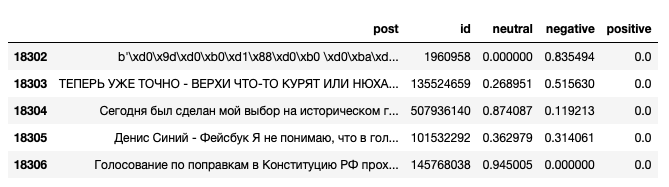Let’s examine the most negative posts:

``df_posts[df_posts.negative > 0.9]``Now, let’s add data about the authors of these posts by merging two tables together on the id column.

``````vk_users = client.execute('SELECT * FROM vk_users')
vk_user_ids_list = []
vk_user_sex_list = []
vk_user_city_list = []
vk_user_age_list = []
for user in vk_users:
vk_user_ids_list.append(user)
vk_user_sex_list.append(user)
vk_user_city_list.append(user)
vk_user_age_list.append(user)
df_users = pd.DataFrame()
df_users['id'] = vk_user_ids_list
df_users['sex'] = vk_user_sex_list
df_users['city'] = vk_user_city_list
df_users['age'] = vk_user_age_list
df = df_posts.merge(df_users, on='id')``````

And the table now looks the following: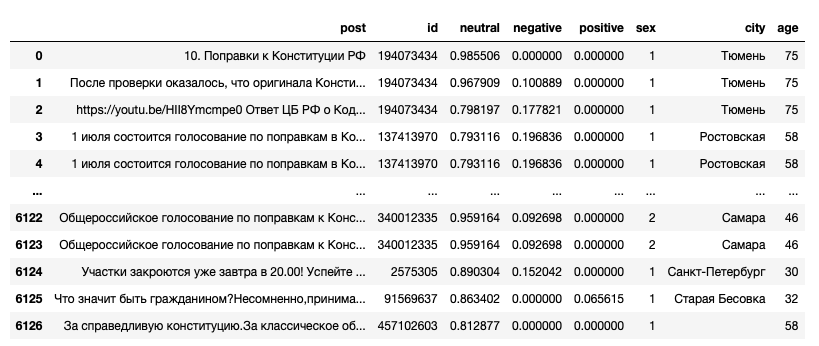## Analysing data with Plotly

Check out our previous article on data visualization with Plotly: Building an interactive waterfall chart in Python

Let’s find the percentage of posts for each group: positive, negative, neutral. Iterate through these three columns and calculate the values more than zero for each data point. Then do the same for different age categories and gender.

According to our chart, 45% of recent user posts relevant to the keyword “constitution” have a negative meaning, while the other 52% are neutral. Later it’ll be known how different the Internet opinions from the voting results.

It’s noticeable that among the men audience the proportion of positive posts is less than 2%, while for women it’s 3.5%. However, the number of negative posts for each group is almost the same, 47% and 43% respectively.

According to our analysis, posts made by younger audiences between 18-25 years have more positive sentiment, which is 6%. While users under 18 years leave mostly negative posts, this may be because most users under the age of 18 prefer to hide their real age, this makes it difficult to obtain accurate data for such a group.
The proportion of negative posts is almost equal for all groups and accounts for 44%.
As you can see, the data is distributed equally in all three charts. This means that half of all posts relevant to the keyword “constitution” and made by VK users over the past week mostly have a negative sentiment.

No comments    98   1 y   Analytics engineering   data analytics   plotly
Earlier Ctrl + ↓